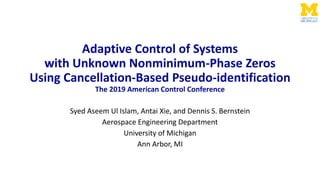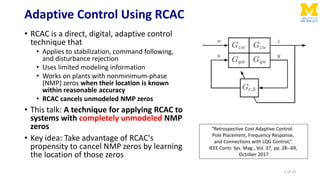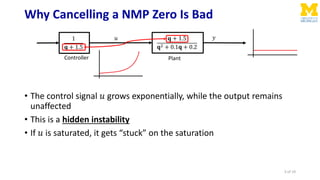Successfully reported this slideshow.

# Adaptive Control of Systems with Unknown Nonminimum-Phase Zeros Using Cancellation-Based Pseudo-Identification×

1 of 19
1 of 19

# Adaptive Control of Systems with Unknown Nonminimum-Phase Zeros Using Cancellation-Based Pseudo-Identification

ACC 2019

ACC 2019

## More Related Content

### Adaptive Control of Systems with Unknown Nonminimum-Phase Zeros Using Cancellation-Based Pseudo-Identification

1. 1. Adaptive Control of Systems with Unknown Nonminimum-Phase Zeros Using Cancellation-Based Pseudo-identification The 2019 American Control Conference Syed Aseem Ul Islam, Antai Xie, and Dennis S. Bernstein Aerospace Engineering Department University of Michigan Ann Arbor, MI
2. 2. Adaptive Control Using RCAC • RCAC is a direct, digital, adaptive control technique that • Applies to stabilization, command following, and disturbance rejection • Uses limited modeling information • Works on plants with nonminimum-phase (NMP) zeros when their location is known within reasonable accuracy • RCAC cancels unmodeled NMP zeros • This talk: A technique for applying RCAC to systems with completely unmodeled NMP zeros • Key idea: Take advantage of RCAC's propensity to cancel NMP zeros by learning the location of those zeros “Retrospective Cost Adaptive Control: Pole Placement, Frequency Response, and Connections with LQG Control,” IEEE Contr. Sys. Mag., Vol. 37, pp. 28--69, October 2017 2 of 19
3. 3. Why Cancelling a NMP Zero Is Bad • The control signal 𝑢 grows exponentially, while the output remains unaffected • This is a hidden instability • If 𝑢 is saturated, it gets “stuck” on the saturation 1 𝐪 + 1.5 𝐪 + 1.5 𝐪2 + 0.1𝐪 + 0.2 𝑢 𝑦 Controller Plant 3 of 19
4. 4. Adaptive Control Using RCAC  RCAC requires limited modeling information embedded in the intercalated target model 𝐺f  𝐺f needs to model the relative degree, NMP zeros (if any), and sign of the leading numerator coefficient of 𝐺𝑧𝑢  Controller order ℓc, adaptation weight 𝑃0 must also be specified  The controller coefficients are initialized to be zero at the start of all numerical examples  Ensures no additional modeling information is used Linear Time-Varying Controller 4 of 19
5. 5. RCAC Does NOT Cancel NMP Zeros When They Are Modeled • 𝐺 𝐪 = 𝐪−1.3 𝐪2−0.7𝐪+0.48 • Broadband disturbance rejection problem • 𝐺f 𝐪 = 𝐪−1.3 𝐪2 • ℓc = 10, 𝑃0 = 100𝐼𝑙 𝜃 Plant NMP zero modeled in 𝐺f Plant NMP zero NOT cancelled by RCAC 5 of 19
6. 6. RCAC Cancels Unmodeled NMP Zeros • 𝐺 𝐪 = 𝐪−1.3 𝐪2−0.7𝐪+0.48 • Broadband disturbance rejection problem • 𝐺f 𝐪 = 1 𝐪 • ℓc = 10, 𝑃0 = 100𝐼𝑙 𝜃 Plant NMP zero not modeled in 𝐺f NMP zero cancelled by controller pole leading to instability 6 of 19
7. 7. RCAC Controller • MIMO Input-Output Controller 𝑢 𝑘 = 𝜙 𝑘 𝜃 𝑘 𝑢 𝑘 = 𝑖=1 ℓc 𝑃𝑖,𝑘 𝑢 𝑘−𝑖 + 𝑖=1 ℓc 𝑄𝑖,𝑘 𝑦 𝑘−𝑖 • Specialization to SISO controller 𝑢 𝑘 = 𝜙 𝑘 𝜃 𝑘 = 𝑢 𝑘 ⋯ 𝑢 𝑘−ℓc 𝑦 𝑘−1 ⋯ 𝑦 𝑘−ℓc 𝑃1,𝑘 ⋮ 𝑃ℓc,𝑘 𝑄1,𝑘 ⋮ 𝑄ℓc,𝑘 𝐺c,𝑘 𝐪 = 𝑄1,𝑘 𝐪ℓc−1 + ⋯ + 𝑄ℓc,𝑘 𝐪ℓc − 𝑃1,𝑘 𝐪ℓc−1 − ⋯ − 𝑃ℓc,𝑘 = 𝑁c,𝑘(𝐪) 𝐷c,𝑘(𝐪) Regressor 7 of 19 Controller Coefficients Transfer Function Representation
8. 8. Retrospective Cost Function • All required modeling information is in the target model 𝑮 𝐟 • We define the retrospective performance variable 𝑧 𝑘 𝜃 ≜ 𝑧 𝑘 − 𝐺f(𝐪) 𝑢 𝑘 − 𝜙 𝑘 𝜃 • Use RLS to minimize the retrospective cost function 𝐽 𝑘 𝜃 ≜ 𝑖=1 𝑘 𝜆 𝑘−𝑖 𝑧𝑖 T 𝜃 𝑅 𝑧 𝑧𝑖 𝜃 + 𝜆 𝑘 𝜃 − 𝜃0 T 𝑃0 −1 𝜃 − 𝜃0 minimizing 𝐽 𝑘 𝜃 ⟹ 𝑧 𝑘 ≈ 𝐺f(𝐪) 𝑢 𝑘( 𝜃) • Updated controller coefficients: 𝜃 𝑘+1 = 𝜃opt,𝑘 Target Model All Modeling Information Past u data Controller coefficients to be re-optimized Past y and u data 8 of 19 Tuning Hyperparameter: Controls adaptation rate Forgetting Factor
9. 9. Recursive Least Squares (RLS) for RCAC 𝑧 𝑘 𝜃 ≜ 𝑧 𝑘 − 𝐺f(𝐪) 𝑢 𝑘 − 𝜙 𝑘 𝜃 𝐽 𝑘 𝜃 ≜ 𝑖=1 𝑘 𝜆 𝑘−𝑖 𝑧𝑖 T 𝜃 𝑅 𝑧 𝑧𝑖 𝜃 + 𝜆 𝑘 𝜃 − 𝜃0 T 𝑃0 −1 𝜃 − 𝜃0 𝜃 𝑘+1 ≜ argmin 𝜃 𝐽 𝑘 𝜃 𝑃𝑘+1 = 1 𝜆 𝑃𝑘 − 1 𝜆 𝑃𝑘Φ 𝑘 T 𝑁T 𝜆𝑅 𝑧 −1 + 𝑁Φ 𝑘 𝑃𝑘Φ 𝑘 T 𝑁T −1 𝑁Φ 𝑘 𝑃𝑘 𝜃 𝑘+1 = 𝜃 𝑘 − 𝑃𝑘Φ 𝑘 T 𝑁T 𝑅 𝑧 𝑁Φ 𝑘 𝜃 𝑘 + 𝑧 𝑘 − 𝑁 𝑈 𝑘 𝑙 𝑧 × 𝑙 𝑧 inverse𝑙 𝜃 × 𝑙 𝜃 matrix 𝑛f 𝑙 𝑢 × 𝑙 𝜃 data buffer 𝑛f 𝑙 𝑢 × 1 data buffer 𝑙 𝑧 × 𝑛f 𝑙 𝑢 filter matrix 𝑙 𝜃 × 1 controller coefficient vector 9 of 19
10. 10. How RCAC Works and Why It Cancels Unmodeled NMP Zeros • RCAC drives the retrospective performance variable to zero 𝑧 𝑘 𝜃 ≜ 𝑧 𝑘 − 𝐺f 𝐪 𝑢 𝑘 where 𝑢 𝑘 ≜ 𝑢 𝑘 − 𝜙 𝑘 𝜃 • Minimization of 𝑧 𝑘 implies 𝑧 𝑘 ≈ 𝐺f 𝐪 𝑢 𝑘( 𝜃) Minimization of 𝑧 “drives” 𝐺 𝑧 𝑢,𝑘 to 𝐺f 𝐺c,𝑘 = 𝑁c,𝑘 𝐷c,𝑘 𝑁𝑧𝑢 ≠ 𝑁𝑦𝑢 NMP zeros unmodeled by 𝐺f may be cancelled by closed-loop poles 𝑁𝑧𝑢 = 𝑁𝑦𝑢 NMP zeros unmodeled by 𝐺f may be cancelled by controller poles 𝐺 𝑧 𝑢,𝑘 𝐪 = 𝑁𝑧𝑢(𝐪)𝐪ℓc 𝐷 𝐪 𝐷c,𝑘 𝐪 − 𝑁𝑦𝑢 𝐪 𝑁c,𝑘(𝐪) ≈ 𝑁f 𝐪 𝑛f 10 of 19
11. 11. RCAC Cancels Unmodeled NMP Zeros (with saturation) • 𝐺 𝐪 = 𝐪−1.3 𝐪2−0.7𝐪+0.48 • Broadband disturbance rejection problem • 𝐺f 𝐪 = 1 𝐪 • ℓc = 10, 𝑃0 = 100𝐼𝑙 𝜃 NMP zero cancelled by controller. Coefficients converge 𝑢 saturated at ±20 𝑢 “rides the saturation” Bad performance! 11 of 19
12. 12. Cancellation-Based Pseudo-Identification • Idea: Utilize RCAC’s propensity to cancel unmodeled NMP zeros to LEARN the NMP zeros of a system • Set 𝐺f = 1 𝐪 (models plant relative degree and sign) • Select control saturation level 𝛼 • If saturation has been activated at least 𝑛 𝛼,max times, and the controller poles have converged, then model all unstable controller poles as zeros in 𝐺f, and reset controller update equations • Formally, If 𝑘 > 2ℓc AND 𝑛 𝛼,𝑘 > 𝑛 𝛼,max AND 𝜃den,𝑘 − 1 ℓc 𝑖=𝑘−ℓc 𝑘 𝜃den,𝑖 < 𝜀 Then model all unstable poles (SR>1.05) of 𝐺c,𝑘 in 𝐺f, and reset 𝑃𝑘 = 𝑃0, 𝜃 𝑘 = 0𝑙 𝜃 Hyperparameters to choose: • ℓc • 𝑃0 • 𝛼 • 𝑛 𝛼,max • 𝜀 The plant is assumed to be asymptotically stable with known relative degree, and leading numerator coefficient sign. The NMP zeros of the plant are unknown. 12 of 19
13. 13. Example 1: Multi-Step Command Following with 1 Real NMP Zero • 𝐺 𝐪 = 𝐪−1.4 𝐪2−0.7𝐪+0.61 Controller pole close to NMP zero at 𝑘 = 64. Subsequently, modeled as zero in 𝐺f. For ALL examples • ℓc = 16 • 𝑃0 = 10𝐼𝑙 𝜃 • 𝛼 = 4 • 𝑛 𝛼,max = 10 • 𝜀 = 0.01 • Sensor noise 𝑣 𝑘~𝑁(0,0.012 ) 13 of 19
14. 14. Example 2: Harmonic Command Following with 2 Complex NMP Zeros • 𝐺 𝐪 = (𝐪−0.43)(𝐪2−2.1𝐪+1.26) (𝐪−0.1)(𝐪−0.2)(𝐪2−1.2𝐪+0.61) Controller poles close to NMP zeros at 𝑘 = 99. Subsequently, modeled as zeros in 𝐺f.𝑟𝑘 = sin 0.13𝑘 For ALL examples • ℓc = 16 • 𝑃0 = 10𝐼𝑙 𝜃 • 𝛼 = 4 • 𝑛 𝛼,max = 10 • 𝜀 = 0.01 • Sensor noise 𝑣 𝑘~𝑁(0,0.012 ) 14 of 19
15. 15. Example 3: Harmonic Disturbance Rejection with 3 NMP Zeros: 1 Negative and 2 Complex • 𝐺 𝐪 = (𝐪+1.4)(𝐪2−1.6𝐪+1.13) (𝐪−0.05)(𝐪−0.25)(𝐪2−𝐪+0.61) Controller poles close to NMP zeros at 𝑘 = 87. Subsequently, modeled as zeros in 𝐺f. 𝑑 𝑘 = 0.1sin 0.234𝑘 For ALL examples • ℓc = 16 • 𝑃0 = 10𝐼𝑙 𝜃 • 𝛼 = 4 • 𝑛 𝛼,max = 10 • 𝜀 = 0.01 • Sensor noise 𝑣 𝑘~𝑁(0,0.012 ) 15 of 19
16. 16. Example 4: Broadband Disturbance Rejection with 4 Complex NMP Zeros • 𝐺 𝐪 = (𝐪2−2.2𝐪+1.37)(𝐪2−1.1𝐪+1.21) (𝐪−0.5)(𝐪2−1.6𝐪+0.76)(𝐪2−0.5𝐪+0.93) For ALL examples • ℓc = 16 • 𝑃0 = 10𝐼𝑙 𝜃 • 𝛼 = 4 • 𝑛 𝛼,max = 10 • 𝜀 = 0.01 • Sensor noise 𝑣 𝑘~𝑁(0,0.012 ) Controller poles close to NMP zeros at 𝑘 = 631. Subsequently, modeled as zeros in 𝐺f. 𝑑 𝑘~𝑁(0,0.12 ) 16 of 19
17. 17. Conclusions and Future Work • Idea: Let the adaptive control attempt to cancel the NMP zeros and use that weakness to learn • A heuristic cancellation-based pseudo-identification technique for adaptive control of NMP plants with UNKNOWN NMP zeros was demonstrated. • The method exploits RCAC’s propensity to cancel unmodeled NMP zeros. • This technique was demonstrated for step and harmonic command following, and harmonic and broadband disturbance rejection. • Plants with up to four unknown NMP zeros were considered. Future work: • Extend this method to unstable systems and MIMO systems 17 of 19
18. 18. Related Talks at ACC 2019 Wednesday • “Adaptive Feedback Noise Control for Wide, Square, and Tall Systems,” A. Xie and D. S. Bernstein, 11:20-11:40, WeA12.5, Room 403 Friday • “Deadbeat Input Reconstruction for Discrete-Time Linear Time-Varying Systems,” A. Ansari and D. S. Bernstein, 13:30-13:50, FrB16.1 , Room 407 • “A Modified RLS Algorithm with Forgetting and Bounded Covariance,” A. Bruce and D. S. Bernstein, 16:00-16:20, FrC04.1, Franklin 4 • “Satellite Drag Estimation Using Retrospective Cost Input Estimation,” A. Ansari and D. S. Bernstein, 17:20-17:40, FrC16.5, Room 407 18 of 19
19. 19. RCAC Does NOT need an exact model of the NMP zero • 𝐺 𝐪 = 𝐪−1.3 𝐪2−0.7𝐪+0.48 • 𝐺f 𝐪 = 𝐪−𝛼 𝑧 𝐪2 Location of plant NMP zero • NMP zero location in 𝐺f is varied. • RCAC works for range of erroneously modeled NMP zeros. • RCAC is most robust to 𝑃0 if the NMP zero in 𝐺f exactly models the NMP zero of the plant. Largest 𝑃0 for which RCAC does not cancel NMP zero 19 of 19

### Editor's Notes

• Perhaps move this one much earlier
• Note that P0 is for RLS to be explained
• Point out forgetting lambda